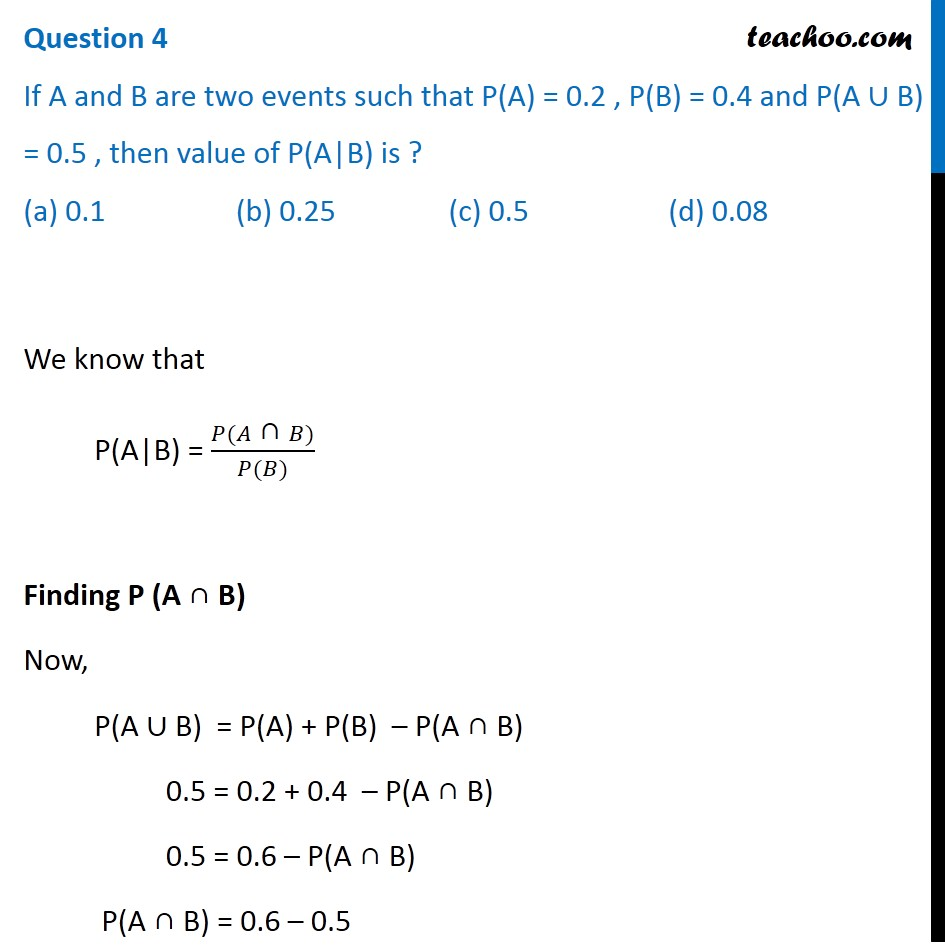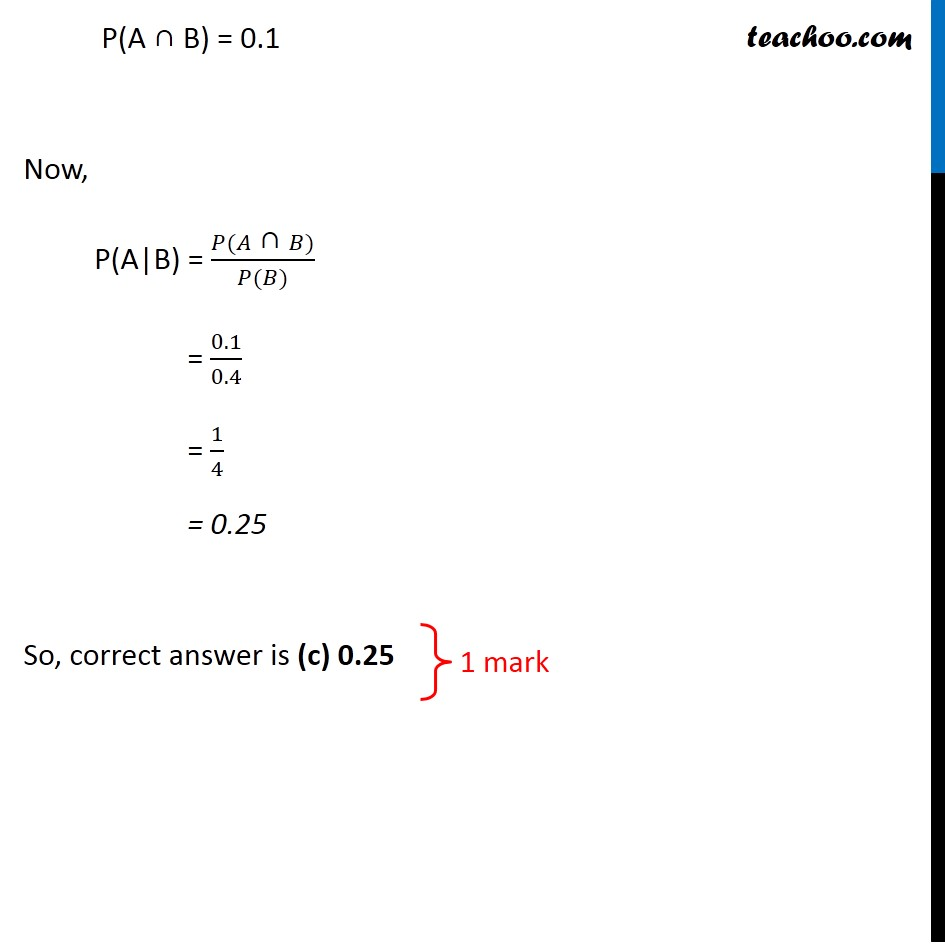CBSE Class 12 Sample Paper for 2020 Boards

Class 12
Solutions of Sample Papers and Past Year Papers - for Class 12 Boards

## (a) 0.1             (b) 0.25             (c) 0.5             (d) 0.08Learn in your speed, with individual attention - Teachoo Maths 1-on-1 Class

### Transcript

Question 4 If A and B are two events such that P(A) = 0.2 , P(B) = 0.4 and P(A ∪ B) = 0.5 , then value of P(A|B) is ? (a) 0.1 (b) 0.25 (c) 0.5 (d) 0.08 We know that P(A|B) = (𝑃(𝐴" ∩ " 𝐵))/(𝑃(𝐵)) Finding P (A ∩ B) Now, P(A ∪ B) = P(A) + P(B) – P(A ∩ B) 0.5 = 0.2 + 0.4 – P(A ∩ B) 0.5 = 0.6 – P(A ∩ B) P(A ∩ B) = 0.6 – 0.5 P(A ∩ B) = 0.1 Now, P(A|B) = (𝑃(𝐴" ∩ " 𝐵))/(𝑃(𝐵)) = 0.1/0.4 = 1/4 = 0.25 So, correct answer is (c) 0.25 1 mark